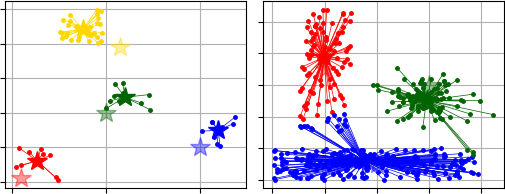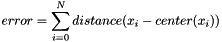pyclustering.cluster.kmeans.kmeans Class Reference

Class implements K-Means clustering algorithm. More...

## Public Member Functions

def __init__ (self, data, initial_centers, tolerance=0.001, ccore=True, kwargs)
Constructor of clustering algorithm K-Means. More...

def process (self)
Performs cluster analysis in line with rules of K-Means algorithm. More...

def predict (self, points)
Calculates the closest cluster to each point. More...

def get_clusters (self)
Returns list of allocated clusters, each cluster contains indexes of objects in list of data. More...

def get_centers (self)
Returns list of centers of allocated clusters. More...

def get_total_wce (self)
Returns sum of metric errors that depends on metric that was used for clustering (by default SSE - Sum of Squared Errors). More...

def get_cluster_encoding (self)
Returns clustering result representation type that indicate how clusters are encoded. More...

## Detailed Description

Class implements K-Means clustering algorithm.

K-Means clustering aims to partition n observations into k clusters in which each observation belongs to the cluster with the nearest mean, serving as a prototype of the cluster. This results in a partitioning of the data space into Voronoi cells.

K-Means clustering results depend on initial centers. Algorithm K-Means++ can used for initialization of initial centers - see module 'pyclustering.cluster.center_initializer'.

CCORE implementation (C/C++ part of the library) of the algorithm performs parallel processing to ensure maximum performance.

Implementation based on the paper .K-Means clustering results. At the left - 'Simple03.data' sample, at the right - 'Lsun.data' sample.

Example #1 - Clustering using K-Means++ for center initialization:

from pyclustering.cluster.kmeans import kmeans, kmeans_visualizer
from pyclustering.cluster.center_initializer import kmeans_plusplus_initializer
from pyclustering.samples.definitions import FCPS_SAMPLES
# Load list of points for cluster analysis.
# Prepare initial centers using K-Means++ method.
initial_centers = kmeans_plusplus_initializer(sample, 2).initialize()
# Create instance of K-Means algorithm with prepared centers.
kmeans_instance = kmeans(sample, initial_centers)
# Run cluster analysis and obtain results.
kmeans_instance.process()
clusters = kmeans_instance.get_clusters()
final_centers = kmeans_instance.get_centers()
# Visualize obtained results
kmeans_visualizer.show_clusters(sample, clusters, final_centers)

Example #2 - Clustering using specific distance metric, for example, Manhattan distance:

# prepare input data and initial centers for cluster analysis using K-Means
# create metric that will be used for clustering
manhattan_metric = distance_metric(type_metric.MANHATTAN)
# create instance of K-Means using specific distance metric:
kmeans_instance = kmeans(sample, initial_centers, metric=manhattan_metric)
# run cluster analysis and obtain results
kmeans_instance.process()
clusters = kmeans_instance.get_clusters()
center_initializer

Definition at line 272 of file kmeans.py.

## ◆ __init__()

 def pyclustering.cluster.kmeans.kmeans.__init__ ( self, data, initial_centers, tolerance = 0.001, ccore = True, kwargs )

Constructor of clustering algorithm K-Means.

Center initializer can be used for creating initial centers, for example, K-Means++ method.

Parameters
 [in] data (array_like): Input data that is presented as array of points (objects), each point should be represented by array_like data structure. [in] initial_centers (array_like): Initial coordinates of centers of clusters that are represented by array_like data structure: [center1, center2, ...]. [in] tolerance (double): Stop condition: if maximum value of change of centers of clusters is less than tolerance then algorithm stops processing. [in] ccore (bool): Defines should be CCORE library (C++ pyclustering library) used instead of Python code or not. [in] **kwargs Arbitrary keyword arguments (available arguments: 'observer', 'metric', 'itermax').

Keyword Args:

• observer (kmeans_observer): Observer of the algorithm to collect information about clustering process on each iteration.
• metric (distance_metric): Metric that is used for distance calculation between two points (by default euclidean square distance).
• itermax (uint): Maximum number of iterations that is used for clustering process (by default: 200).
center_initializer

Definition at line 333 of file kmeans.py.

## ◆ get_centers()

 def pyclustering.cluster.kmeans.kmeans.get_centers ( self )

Returns list of centers of allocated clusters.

process()
get_clusters()

Definition at line 479 of file kmeans.py.

## ◆ get_cluster_encoding()

 def pyclustering.cluster.kmeans.kmeans.get_cluster_encoding ( self )

Returns clustering result representation type that indicate how clusters are encoded.

Returns
(type_encoding) Clustering result representation.
get_clusters()

Definition at line 508 of file kmeans.py.

## ◆ get_clusters()

 def pyclustering.cluster.kmeans.kmeans.get_clusters ( self )

Returns list of allocated clusters, each cluster contains indexes of objects in list of data.

process()
get_centers()

Definition at line 467 of file kmeans.py.

## ◆ get_total_wce()

 def pyclustering.cluster.kmeans.kmeans.get_total_wce ( self )

Returns sum of metric errors that depends on metric that was used for clustering (by default SSE - Sum of Squared Errors).

Sum of metric errors is calculated using distance between point and its center:process()
get_clusters()

Definition at line 494 of file kmeans.py.

## ◆ predict()

 def pyclustering.cluster.kmeans.kmeans.predict ( self, points )

Calculates the closest cluster to each point.

Parameters
 [in] points (array_like): Points for which closest clusters are calculated.
Returns
(list) List of closest clusters for each point. Each cluster is denoted by index. Return empty collection if 'process()' method was not called.

Definition at line 442 of file kmeans.py.

## ◆ process()

 def pyclustering.cluster.kmeans.kmeans.process ( self )

Performs cluster analysis in line with rules of K-Means algorithm.

Returns
(kmeans) Returns itself (K-Means instance).